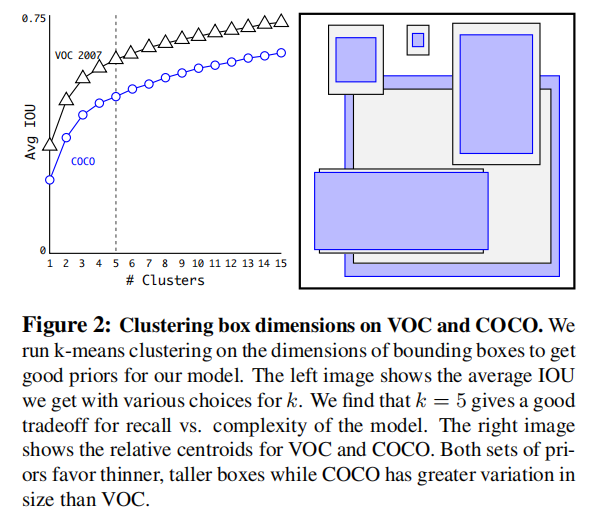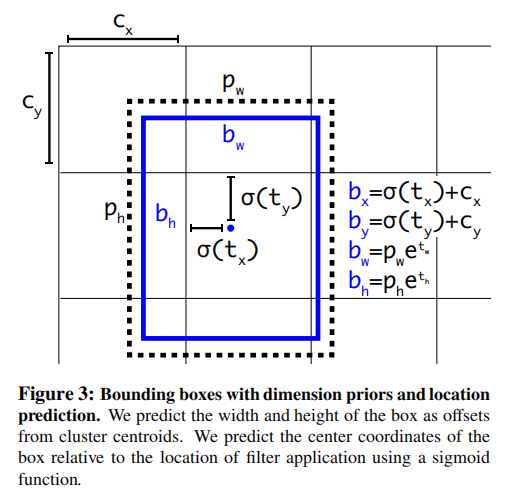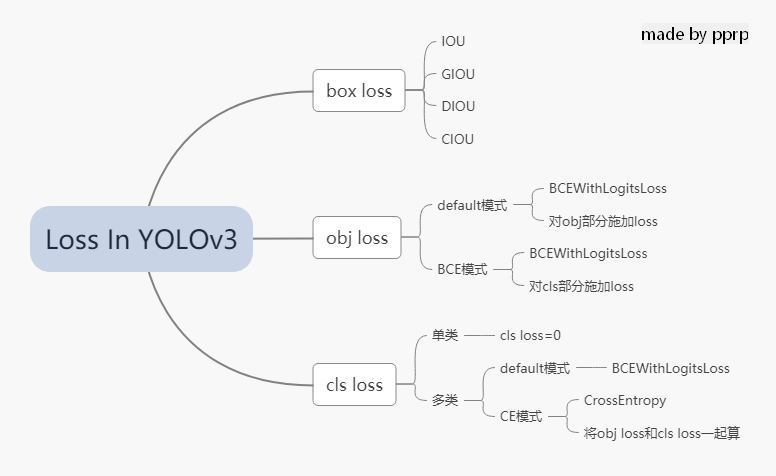# 【从零开始学习YOLOv3】8. YOLOv3中Loss部分计算¶

YOLOv1是一个anchor-free的，从YOLOv2开始引入了Anchor，在VOC2007数据集上将mAP提升了10个百分点。YOLOv3也继续使用了Anchor，本文主要讲ultralytics版YOLOv3的Loss部分的计算, 实际上这部分loss和原版差距非常大，并且可以通过arc指定loss的构建方式, 如果想看原版的loss可以在下方release的v6中下载源码。

Github release: https://github.com/ultralytics/yolov3/releases

## 1. Anchor¶

Faster R-CNN中Anchor的大小和比例是由人手工设计的，可能并不贴合数据集，有可能会给模型性能带来负面影响。YOLOv2和YOLOv3则是通过聚类算法得到最适合的k个框。聚类距离是通过IoU来定义，IoU越大，边框距离越近。

d(box,centroid)=1-IoU(box,centroid)

Anchor越多，平均IoU会越大，效果越好，但是会带来计算量上的负担，下图是YOLOv2论文中的聚类数量和平均IoU的关系图，在YOLOv2中选择了5个anchor作为精度和速度的平衡。## 2. 偏移公式¶

\left\{ \begin{aligned} x=&(t_x\times w_a)+x_a\\ y=&(t_y\times h_a)+y_a \end{aligned} \right.

\left\{ \begin{aligned} b_x&=\sigma(t_x)+c_x\\ b_y&=\sigma(t_y)+c_y\\ b_w&=p_we^{t_w}\\ b_h&=p_he^{t_h}\\ \sigma(t_o)&=Pr(object)\times IOU(b,object) \end{aligned} \right.• $c_x$$c_y$分别代表中心点所处区域的左上角坐标。
• $p_w$$p_h$分别代表Anchor的宽和高。
• $\sigma(t_x)$$\sigma(t_y)$分别代表预测框中心点和左上角的距离，$\sigma$代表sigmoid函数，将偏移量限制在当前grid中，有利于模型收敛。
• $t_w$$t_h$代表预测的宽高偏移量，Anchor的宽和高乘上指数化后的宽高，对Anchor的长宽进行调整。

• $\sigma(t_o)$是置信度预测值，是当前框有目标的概率乘以bounding box和ground truth的IoU的结果

## 3. Loss¶

YOLOv3中有一个参数是ignore_thresh，在ultralytics版版的YOLOv3中对应的是train.py文件中的iou_t参数（默认为0.225）。

• 如果一个预测框与所有的Ground Truth的最大IoU<ignore_thresh时，那这个预测框就是负样本

• 如果Ground Truth的中心点落在一个区域中，该区域就负责检测该物体。将与该物体有最大IoU的预测框作为正样本（注意这里没有用到ignore thresh,即使该最大IoU<ignore thresh也不会影响该预测框为正样本）

• 一个是xywh部分带来的误差，也就是bbox带来的loss
• 一个是置信度带来的误差，也就是obj带来的loss
• 最后一个是类别带来的误差，也就是class带来的loss

\begin{aligned} lbox &= \lambda_{coord}\sum_{i=0}^{S^2}\sum_{j=0}^{B}1_{i,j}^{obj}(2-w_i\times h_i)[(x_i-\hat{x_i})^2+(y_i-\hat{y_i})^2+(w_i-\hat{w_i})^2+(h_i-\hat{h_i})^2] \\ lcls &= \lambda_{class}\sum_{i=0}^{S^2}\sum_{j=0}^{B}1_{i,j}^{obj}\sum_{c\in classes}p_i(c)log(\hat{p_i}(c))\\ lobj &= \lambda_{noobj}\sum_{i=0}^{S^2}\sum_{j=0}^{B}1_{i,j}^{noobj}(c_i-\hat{c_i})^2+\lambda_{obj}\sum_{i=0}^{S^2}\sum_{j=0}^{B}1_{i,j}^{obj}(c_i-\hat{c_i})^2\\ loss &= lbox + lobj + lcls \end{aligned}

S: 代表grid size, $S^2$代表13x13,26x26, 52x52

B: box

$1_{i,j}^{obj}$: 如果在i,j处的box有目标，其值为1，否则为0

$1_{i,j}^{noobj}$: 如果在i,j处的box没有目标，其值为1，否则为0

BCE（binary cross entropy）具体计算公式如下：

BCE(\hat{c_i},c_i)=-\hat{c_i}\times log(c_i)-(1-\hat{c_i})\times log(1-c_i)

1. lbox部分

IoU=\frac{|A\cap B|}{|A\cup B|}

GIoU=IoU-\frac{|A_c-U|}{|A_c|}

yolov3中提供了IoU、GIoU、DIoU和CIoU等计算方式，以GIoU为例：

if GIoU:  # Generalized IoU https://arxiv.org/pdf/1902.09630.pdf
c_area = cw * ch + 1e-16  # convex area
return iou - (c_area - union) / c_area  # GIoU


giou = bbox_iou(pbox.t(), tbox[i],
x1y1x2y2=False, GIoU=True)
lbox += (1.0 - giou).sum() if red == 'sum' else (1.0 - giou).mean()


2. lobj部分

lobj代表置信度，即该bounding box中是否含有物体的概率。在yolov3代码中obj loss可以通过arc来指定，有两种模式：

BCEobj = nn.BCEWithLogitsLoss(pos_weight=ft([h['obj_pw']]), reduction=red)
if 'default' in arc:  # separate obj and cls
lobj += BCEobj(pi[..., 4], tobj)  # obj loss
# pi[...,4]对应的是该框中含有目标的置信度，和giou计算BCE
# 相当于将obj loss和cls loss分开计算


BCE = nn.BCEWithLogitsLoss(reduction=red)
elif 'BCE' in arc:  # unified BCE (80 classes)
t = torch.zeros_like(pi[..., 5:])  # targets
if nb:
t[b, a, gj, gi, tcls[i]] = 1.0 # 对应正样本class置信度设置为1
lobj += BCE(pi[..., 5:], t)#pi[...,5:]对应的是所有的class


3. lcls部分

BCEcls = nn.BCEWithLogitsLoss(pos_weight=ft([h['cls_pw']]), reduction=red)
# cls loss 只计算多类之间的loss,单类不进行计算
if 'default' in arc and model.nc > 1:
t = torch.zeros_like(ps[:, 5:])  # targets
t[range(nb), tcls[i]] = 1.0 # 设置对应class为1
lcls += BCEcls(ps[:, 5:], t)  # 使用BCE计算分类loss


CE = nn.CrossEntropyLoss(reduction=red)
elif 'CE' in arc:  # unified CE (1 background + 80 classes)
t = torch.zeros_like(pi[..., 0], dtype=torch.long)  # targets
if nb:
t[b, a, gj, gi] = tcls[i] + 1 # 由于cls是从零开始计数的，所以+1
lcls += CE(pi[..., 4:].view(-1, model.nc + 1), t.view(-1))
# 这里将obj loss和cls loss一起计算，使用CrossEntropy Loss## 4. 代码¶

ultralytics版版的yolov3的loss已经和论文中提出的部分大相径庭了，代码中很多地方地方是来自作者的经验。另外，这里读的代码是2020年2月份左右作者发布的版本，关注这个库的人会知道，作者更新速度非常快，在笔者写这篇文章的时候，loss也出现了大幅改动，添加了label smoothing等新的机制，去掉了通过arc来调整loss的机制，简化了loss部分。

• 单类数据集class=1
• batch size=2
• 模型是yolov3.cfg

1. 正负样本选取部分

• 如果一个预测框与所有的Ground Truth的最大IoU<ignore_thresh时，那这个预测框就是负样本

• 如果Ground Truth的中心点落在一个区域中，该区域就负责检测该物体。将与该物体有最大IoU的预测框作为正样本（注意这里没有用到ignore thresh,即使该最大IoU<ignore thresh也不会影响该预测框为正样本）

def build_targets(model, targets):
# targets = [image, class, x, y, w, h]
# 这里的image是一个数字，代表是当前batch的第几个图片
# x,y,w,h都进行了归一化，除以了宽或者高

nt = len(targets)

tcls, tbox, indices, av = [], [], [], []

multi_gpu = type(model) in (nn.parallel.DataParallel,
nn.parallel.DistributedDataParallel)

reject, use_all_anchors = True, True
for i in model.yolo_layers:
# yolov3.cfg中有三个yolo层，这部分用于获取对应yolo层的grid尺寸和anchor大小
# ng 代表num of grid (13,13) anchor_vec [[x,y],[x,y]]
# 注意这里的anchor_vec: 假如现在是yolo第一个层(downsample rate=32)
# 这一层对应anchor为：[116, 90], [156, 198], [373, 326]
# anchor_vec实际值为以上除以32的结果：[3.6,2.8],[4.875,6.18],[11.6,10.1]
# 原图 416x416 对应的anchor为 [116, 90]
# 下采样32倍后 13x13 对应的anchor为 [3.6,2.8]
if multi_gpu:
ng = model.module.module_list[i].ng
anchor_vec = model.module.module_list[i].anchor_vec
else:
ng = model.module_list[i].ng,
anchor_vec = model.module_list[i].anchor_vec

# iou of targets-anchors
# targets中保存的是ground truth
t, a = targets, []

gwh = t[:, 4:6] * ng

if nt:  # 如果存在目标
# anchor_vec: shape = [3, 2] 代表3个anchor
# gwh: shape = [2, 2] 代表 2个ground truth
# iou: shape = [3, 2] 代表 3个anchor与对应的两个ground truth的iou
iou = wh_iou(anchor_vec, gwh)  # 计算先验框和GT的iou

if use_all_anchors:
na = len(anchor_vec)  # number of anchors
a = torch.arange(na).view(
(-1, 1)).repeat([1, nt]).view(-1)  # 构造 3x2 -> view到6
# a = [0,0,1,1,2,2]
t = targets.repeat([na, 1])
# targets: [image, cls, x, y, w, h]
# 复制3个: shape[2,6] to shape[6,6]
gwh = gwh.repeat([na, 1])
# gwh shape:[6,2]
else:  # use best anchor only
iou, a = iou.max(0)  # best iou and anchor
# 取iou最大值是darknet的默认做法，返回的a是下角标

# reject anchors below iou_thres (OPTIONAL, increases P, lowers R)
if reject:
# 在这里将所有阈值小于ignore thresh的去掉
j = iou.view(-1) > model.hyp['iou_t']
# iou threshold hyperparameter
t, a, gwh = t[j], a[j], gwh[j]

# Indices
b, c = t[:, :2].long().t()  # target image, class
# 取的是targets[image, class, x,y,w,h]中 [image, class]

gxy = t[:, 2:4] * ng  # grid x, y

gi, gj = gxy.long().t()  # grid x, y indices
# 注意这里通过long将其转化为整形，代表格子的左上角

indices.append((b, a, gj, gi))
# indice结构体保存内容为：
'''
b: 一个batch中的角标
a: 代表所选中的正样本的anchor的下角标
gj, gi: 代表所选中的grid的左上角坐标
'''
# Box
gxy -= gxy.floor()  # xy
# 现在gxy保存的是偏移量，是需要YOLO进行拟合的对象
tbox.append(torch.cat((gxy, gwh), 1))  # xywh (grids)
# 保存对应偏移量和宽高（对应13x13大小的）
av.append(anchor_vec[a])  # anchor vec
# av 是anchor vec的缩写，保存的是匹配上的anchor的列表

# Class
tcls.append(c)
# tcls用于保存匹配上的类别列表
if c.shape:  # if any targets
assert c.max() < model.nc, 'Model accepts %g classes labeled from 0-%g, however you labelled a class %g. ' \
'See https://github.com/ultralytics/yolov3/wiki/Train-Custom-Data' % (
model.nc, model.nc - 1, c.max())
return tcls, tbox, indices, av


• 将ground truth和anchor进行匹配，得到iou
• 然后有两个方法匹配：
• 使用yolov3原版的匹配机制，仅仅选择iou最大的作为正样本
• 使用ultralytics版版yolov3的默认匹配机制，use_all_anchors=True的时候，选择所有的匹配对
• 对以上匹配的部分在进行筛选，对应原版yolo中ignore_thresh部分，将以上匹配到的部分中iou<ignore_thresh的部分筛选掉。
• 最后将匹配得到的内容返回到compute_loss函数中。

2. loss计算部分

def compute_loss(p, targets, model):
# p: (bs, anchors, grid, grid, classes + xywh)
# predictions, targets, model
ft = torch.cuda.FloatTensor if p.is_cuda else torch.Tensor
lcls, lbox, lobj = ft(), ft(), ft()
tcls, tbox, indices, anchor_vec = build_targets(model, targets)
'''
以yolov3为例，有三个yolo层
tcls: 一个list保存三个tensor,每个tensor中有6(2个gtx3个anchor)个代表类别的数字
tbox: 一个list保存三个tensor,每个tensor形状[6,4],6(2个gtx3个anchor)个bbox
indices: 一个list保存三个tuple,每个tuple中保存4个tensor：
分别代表        b: 一个batch中的角标
a: 代表所选中的正样本的anchor的下角标
gj, gi: 代表所选中的grid的左上角坐标
anchor_vec: 一个list保存三个tensor,每个tensor形状[6,2],
6(2个gtx3个anchor)个anchor,注意大小是相对于13x13feature map的anchor大小
'''

h = model.hyp  # hyperparameters
arc = model.arc  # # (default, uCE, uBCE) detection architectures
# 具体使用的损失函数是通过arc参数决定的
red = 'sum'  # Loss reduction (sum or mean)

# Define criteria
BCEcls = nn.BCEWithLogitsLoss(pos_weight=ft([h['cls_pw']]), reduction=red)
BCEobj = nn.BCEWithLogitsLoss(pos_weight=ft([h['obj_pw']]), reduction=red)
#BCEWithLogitsLoss = sigmoid + BCELoss
BCE = nn.BCEWithLogitsLoss(reduction=red)
CE = nn.CrossEntropyLoss(reduction=red)  # weight=model.class_weights

# class label smoothing https://arxiv.org/pdf/1902.04103.pdf eqn 3
# cp, cn = smooth_BCE(eps=0.0)
# 这是最新的版本中提供了label smoothing的功能，只能用在多类问题

if 'F' in arc:  # add focal loss
g = h['fl_gamma']
BCEcls, BCEobj, BCE, CE = FocalLoss(BCEcls, g), FocalLoss(
BCEobj, g), FocalLoss(BCE, g), FocalLoss(CE, g)
# focal loss可以用在cls loss或者obj loss

# Compute losses
np, ng = 0, 0  # number grid points, targets
# np这个命名真的迷，建议改一下和numpy缩写重复
for i, pi in enumerate(p):  # layer index, layer predictions
# 在yolov3中，p有三个yolo layer的输出pi
# 形状为:(bs, anchors, grid, grid, classes + xywh)
b, a, gj, gi = indices[i]  # image, anchor, gridy, gridx
tobj = torch.zeros_like(pi[..., 0])
# tobj = target obj, 形状为(bs, anchors, grid, grid)
np += tobj.numel() # 返回tobj中元素个数

# Compute losses
nb = len(b)
if nb:
ng += nb # number of targets 用于最后算平均loss
# (bs, anchors, grid, grid, classes + xywh)
ps = pi[b, a, gj, gi] # 即找到了对应目标的classes+xywh，形状为[6(2x3),6]

# GIoU
pxy = torch.sigmoid(
ps[:, 0:2] # 将x,y进行sigmoid
)  # pxy = pxy * s - (s - 1) / 2,  s = 1.5  (scale_xy)
pwh = torch.exp(ps[:, 2:4]).clamp(max=1E3) * anchor_vec[i]
# 防止溢出进行clamp操作,乘以13x13feature map对应的anchor
# 这部分和上文中偏移公式是一致的
pbox = torch.cat((pxy, pwh), 1)  # predicted box
# pbox: predicted bbox shape:[6, 4]
giou = bbox_iou(pbox.t(), tbox[i], x1y1x2y2=False,
GIoU=True)  # giou computation
# 计算giou loss， 形状为6
lbox += (1.0 - giou).sum() if red == 'sum' else (1.0 - giou).mean()
# bbox loss直接由giou决定
tobj[b, a, gj, gi] = giou.detach().type(tobj.dtype)
# target obj 用giou取代1，代表该点对应置信度

# cls loss 只计算多类之间的loss,单类不进行计算
if 'default' in arc and model.nc > 1:
t = torch.zeros_like(ps[:, 5:])  # targets
t[range(nb), tcls[i]] = 1.0 # 设置对应class为1
lcls += BCEcls(ps[:, 5:], t)  # 使用BCE计算分类loss

if 'default' in arc:  # separate obj and cls
lobj += BCEobj(pi[..., 4], tobj)  # obj loss
# pi[...,4]对应的是该框中含有目标的置信度，和giou计算BCE
# 相当于将obj loss和cls loss分开计算

elif 'BCE' in arc:  # unified BCE (80 classes)
t = torch.zeros_like(pi[..., 5:])  # targets
if nb:
t[b, a, gj, gi, tcls[i]] = 1.0 # 对应正样本class置信度设置为1
lobj += BCE(pi[..., 5:], t)
#pi[...,5:]对应的是所有的class

elif 'CE' in arc:  # unified CE (1 background + 80 classes)
t = torch.zeros_like(pi[..., 0], dtype=torch.long)  # targets
if nb:
t[b, a, gj, gi] = tcls[i] + 1 # 由于cls是从零开始计数的，所以+1
lcls += CE(pi[..., 4:].view(-1, model.nc + 1), t.view(-1))
# 这里将obj loss和cls loss一起计算，使用CrossEntropy Loss
# 使用对应的权重来平衡，这个参数是作者通过参数搜索（random search）的方法搜索得到的
lbox *= h['giou']
lobj *= h['obj']
lcls *= h['cls']

if red == 'sum':
bs = tobj.shape  # batch size
lobj *= 3 / (6300 * bs) * 2
# 6300 = (10 ** 2 + 20 ** 2 + 40 ** 2) * 3
# 输入为320x320的图片，则存在6300个anchor
# 3代表3个yolo层, 2是一个超参数，通过实验获取
# 如果不想计算的话，可以修改red='mean'
if ng:
lcls *= 3 / ng / model.nc
lbox *= 3 / ng
loss = lbox + lobj + lcls
return loss, torch.cat((lbox, lobj, lcls, loss)).detach()


## 5. 补充¶

torch.nn.BCELoss功能是二分类任务是的交叉熵计算函数，可以认为是CrossEntropy的特例。其分类限定为二分类，y的值必须为{0,1}，input应该是概率分布的形式。在使用BCELoss前一般会先加一个sigmoid激活层，常用在自编码器中。

l_n=-w_n[y_nlog(x_n)+(1-y_n)log(1-x_n)]

$w_n$是每个类别的loss权重，用于类别不均衡问题。

torch.nn.BCEWithLogitsLoss的相当于Sigmoid+BCELoss, 即input会经过Sigmoid激活函数，将input变为概率分布的形式。

l_n=-w_n[y_nlog\sigma(x_n)+(1-y_n)log(1-\sigma(x_n))]